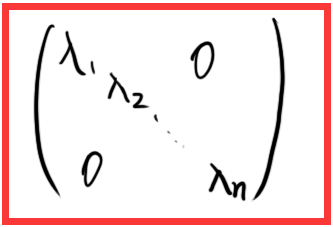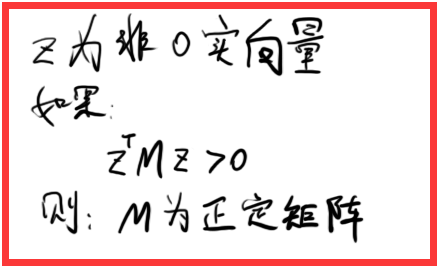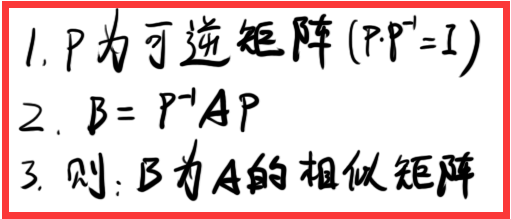• 一般矩阵的相似对角化用它的特征向量组成的矩阵就可以了，为什么实对称矩阵的相似对角化这么特殊呢，名称叫做正交矩阵化，求得特征向量矩阵后还要正交化和单位化使之成为正交矩阵呢？ 答： 对称矩阵也可以用一般的由...


为什么实对称矩阵的相似对角化要用正交矩阵？
一般矩阵的相似对角化用它的特征向量组成的矩阵就可以了，为什么实对称矩阵的相似对角化这么特殊呢，名称叫做正交矩阵化，求得特征向量矩阵后还要正交化和单位化使之成为正交矩阵呢？
答：
对称矩阵也可以用一般的由特征向量组成的非奇异阵做对角化，只不过它有特殊的性质（对称），因此我们就可以考虑特殊的对角化，也就是正交相似对角化。这么做有好处：正交矩阵的逆矩阵很容易求，就是它的转置，不像一般的可逆阵需要半天才能求出来。你想想，如果是一个1000*1000的矩阵求逆，那要多长时间才能做完？但正交矩阵就太容易了，只要转置一下就行了。

一般矩阵，非实对称矩阵，如果它满足相似对角化的条件 那它可不可以正交对角化？
答：能。
因为只有对称矩阵才有这样一个性质：对于不同特征值对应的特征向量，它们互相正交
因此，对于重特征值，则可以通过正交化来获得对应的相互正交的特征向量。再与其他特征值的特征向量一起，构成了n个相互正交的特征向量。
而对于非对称矩阵，虽然对于重特征值时，你可以用同样的正交化方法获得相互正交的同特征值对应特征向量，但是不同特征值对应的特征向量不是正交的，而不同的特征值对应的特征向量用正交化方法是没有意义的（你能算出来，但结果不再是特征向量了，因为不同特征值的特征向量相互线性运算后不再是特征向量），所以没有办法获得N个相互正交的特征向量的。
也许你会问如果是N个相同特征值的非对称矩阵是不是就能够用正交化方法获得N个相互正交的特征向量了，但是对于这种情况，你的矩阵必须是对称的，否则不满足相似对角化条件，即找不到n个线性无关特征向量。


展开全文• 1 ，对称矩阵 ： 定于 ： 1 ，如果 ：矩阵 A = A 的转置 2 ，那么 ：A 为对称矩阵 如图 ： 2 ，对角矩阵 ： ...1 ，主对角线的元素不为 0 ...5 ，对角化 ： ...1 ，如果一个矩阵的像是矩阵是一个...2 ，则称这个过程是对角化
1 ，对称矩阵 ：

定于 ：
1 ，如果 ：矩阵 A = A 的转置
2 ，那么 ：A 为对称矩阵
如图 ：2 ，对角矩阵 ：

定义 ：
1 ，主对角线的元素不为 0
2 ，其他元素都为 0
例如 ：3 ，正定矩阵 ：

定义 ：
1 ，可以让非零实向量乘以他自己的转置 > 0 ，这样的矩阵叫正定矩阵
2 ，理解 ： 把它的方向正过来
如图 ：4 ，相似矩阵 ：

定义 ：5 ，对角化 ：

定义 ：
1 ，如果一个矩阵的像是矩阵是一个对角矩阵
2 ，则称这个过程是对角化


展开全文线性代数
• ## 循环矩阵傅里叶对角化

万次阅读 多人点赞 2016-03-15 14:10:30
“任意循环矩阵可以被傅里叶变换矩阵对角化”这个概念常常出现在论文中，本文对其做简单解释。

All circulant matrices are made diagonal by the Discrete Fourier Transform (DFT), regardless of the generating vector x.
任意循环矩阵可以被傅里叶变换矩阵对角化。

文献中，一般用如下方式表达这一概念：
$X=C(x)=F \cdot diag(\hat{x}) \cdot F^H$
其中$X$是循环矩阵，$\hat{x}$是原向量$x$的傅里叶变换，$F$是傅里叶变换矩阵，上标H表示共轭转置：$X^H=(X^{*})^T$。
换句话说，$X$相似于对角阵，$X$的特征值是$\hat x$的元素。
另一方面，如果一个矩阵能够表示成两个傅里叶矩阵夹一个对角阵的乘积形式，则它是一个循环矩阵。其生成向量是对角元素的傅里叶逆变换：
$F \cdot diag(y) \cdot F^H = C(\mathcal{F}^{-1}(y))$
这个公式初看疑问很多，以下一一讨论。
$X$是什么？
$X$是由原向量$x$生成的循环矩阵。以矩阵尺寸$K=4$为例。
$X=C(x)=\begin{bmatrix} x_1 & x_2 & x_3 & x_4 \\ x_4 & x_1 & x_2 & x_3 \\ x_3 & x_4 & x_1 & x_2 \\ x_2 & x_3 & x_4 & x_1 \\ \end{bmatrix}$
$F$ 是什么？
$F$是离散傅里叶矩阵（DFT matrix），可以用一个复数$\omega = e^{-2\pi i/K}$表示，其中$K$为方阵$F$的尺寸。以$K=4$为例。
$F=\frac{1}{\sqrt{K}} \begin{bmatrix} 1 & 1 & 1 & 1 \\ 1 & \omega & \omega^2 & \omega^3 \\ 1 & \omega^2 & \omega ^4 & \omega^6 \\ 1 & \omega^3 & \omega^6 & \omega^9 \end{bmatrix}$
把$\omega$想象成一个角度为$2\pi/K$的向量，这个矩阵的每一行是这个向量在不断旋转。从上到下，旋转速度越来越快。
之所以称为DFT matrix，是因为一个信号的DFT变换可以用此矩阵的乘积获得：
$\hat{x}=DFT(x)=\sqrt{K}\cdot F \cdot x$
反傅里叶变换也可以通过类似手段得到：
$x=\frac{1}{\sqrt{K}}F^{-1}\hat{x}$
傅里叶矩阵有许多性质：

是对称矩阵，观察$\omega$的规律即可知；
满足$F^HF=FF^H=I$，也就是说它是个酉矩阵（unitary）。可以通过将$F^{H}$展开成$\omega^{H}$验证。

注意：$F$是常数，可以提前计算好，和要处理的$x$无关。
对角化怎么理解？
把原公式两边乘以逆矩阵：
$F^{-1} \cdot X \cdot (F^H)^{-1}=diag(\hat{x})$
利用前述酉矩阵性质：
$左边=F^{H}XF=diag(\hat{x})$
也就是说，矩阵$X$通过相似变换$F$变成对角阵$diag(\hat{x})$，即对循环矩阵$X$进行对角化。
另外，$F^HXF$是矩阵$X$的2D DFT变换。即傅里叶变换可以把循环矩阵对角化。
怎么证明？
可以用构造特征值和特征向量的方法证明（参看这篇论文1的3.1节），此处简单描述。
考察待证明等式的第k列：
$X\cdot f_k=\hat x_k\cdot f_k$
其中$f_k$表示DFT矩阵的第k列，$\hat x_k$表示傅里叶变换的第k个元素。等价于求证：$f_k$和$\hat x_k$是$X$的一对特征向量和特征值。
左边向量的第i个元素为：$left_i = \left[ x^i, f_k \right]$。$x_i$表示把生成向量$x$向右移动i位，$[]$表示内积。
内积只和两个向量的相对位移有关，所以可以把$f_k$向左移动i位：$left_i=\left[ x, f_k^{-i}\right]$。
DFT矩阵列的移位可以通过数乘$\omega$的幂实现：$f_k^i=f_0\cdot \omega^{ik}$。

举例：$K=3$，
$F=\frac{1}{\sqrt{K}} \begin{bmatrix} 1 & 1 & 1\\ 1 & \omega & \omega^2 \\ 1 & \omega^2 & \omega ^4 \end{bmatrix}$

利用$\omega^N=1$.
$f_1\cdot \omega =[1,\omega,\omega^2]\cdot \omega = [\omega, \omega^2,\omega^3] = [\omega, \omega^2, 1]=f_1^{-1}$

$f_1\cdot \omega^2 =[1,\omega,\omega^2]\cdot \omega^2 = [\omega^2, \omega^3,\omega^4] = [\omega^2, 1, \omega]=f_1^{-2}$

于是有：
$left_i= \left [x, f_k \cdot \omega^{ik}\right ]=\left [x, f_k\right ] \cdot \omega^{ik}$
右边的$\hat x = F\cdot x $，考虑到$F$的第k行和第k列相同，$\hat x_k=[f_k,x]$。另外$f_k$的第i个元素为$\omega^{ik}$：
$right_i = \hat x_k\cdot f_{ki}=[f_k^*,x]\cdot \omega^{ki}$
对任意k列的第i个元素有：$left_i=right_i$
更多性质
利用对角化，能推导出循环矩阵的许多性质。
转置
循环矩阵的转置也是一个循环矩阵（可以查看循环矩阵各元素排列证明），其特征值和原特征值共轭。
$X^T=F \cdot diag((\hat{x})^*) \cdot F^H$
可以通过如下方式证明：
$X^T=(F^H)^T\cdot diag(\hat x) F^T$
由于$F$是对称酉矩阵，且已知$X$是实矩阵：
$X^T=F^*\cdot diag(\hat x) F=\left( F^*\cdot diag(\hat x) F\right)^*=F\cdot diag((\hat x)^*) \cdot F^H$
如果原生成向量$x$是对称向量（例如[1,2,3,4,3,2]），则其傅里叶变换为实数，则：
$X^T=C\left( \mathcal F^{-1}(\hat x)^* \right)=C(x)$
卷积
循环矩阵乘向量等价于生成向量的逆序和该向量卷积，可进一步转化为福利也变化相乘。
注意卷积本身即包含逆序操作，另外利用了信号与系统中经典的“时域卷积，频域相乘”。
$\mathcal{F}(Xy) = \mathcal{F}(C(x)y)=\mathcal{F}(\bar x*y)=\mathcal{F}^*(x)\odot \mathcal{F}(y)$
其中$\bar x$表示把$x$的元素倒序排列。星号表示共轭。
相乘
设$C,B$为循环矩阵，其乘积的特征值等于特征值的乘积：
$AB = F\cdot diag(\hat a) \cdot F^H \cdot F \cdot diag(\hat b) \cdot F^H$
$= F\cdot diag(\hat a) \cdot diag(\hat b) \cdot F^H=F\cdot diag(\hat a \odot \hat b) \cdot F^H$
$=C\left( \mathcal F^{-1}(\hat a \odot \hat b)\right)$
乘积也是循环矩阵，其生成向量是原生成向量对位相乘的傅里叶逆变换。
相加
和的特征值等于特征值的和：
$A+B = F\cdot diag(\hat a) \cdot F^H + F \cdot diag(\hat b) \cdot F^H=F\cdot diag(\hat a +\hat b) \cdot F^H$
$=C\left( \mathcal F^{-1} (\hat a +\hat b)\right)=C\left( \mathcal F^{-1} (\hat a )+F^{-1} (\hat b )\right)=C\left( a+b\right)$
和也是循环矩阵，其生成向量是原生成向量的和。
求逆
循环矩阵的逆，等价于将其特征值求逆。
$X^{-1}=F\cdot diag(\hat{x})^{-1}F^H=C\left( \mathcal F^{-1}(diag(\hat{x})^{-1}) \right)$
对角阵求逆等价于对角元素求逆。以下证明：
$F\cdot diag(\hat{x})^{-1}\cdot F^H \cdot F\cdot diag(\hat{x}) \cdot F^H$
$=F\cdot diag(\hat{x})^{-1}\cdot diag(\hat{x}) \cdot F^H=F\cdot F^H=I$
逆也是循环矩阵
有什么用？
该性质可以将循环矩阵的许多运算转换成更简单的运算。例如：
$X^HX=F \cdot diag(\hat{x} \odot \hat{x}^*)\cdot F^H=C\left( \mathcal F^{-1}(\hat{x} \odot \hat{x}^*) \right)$
原始计算量：两个方阵相乘（$O(K^3)$）
转化后的计算量：反向傅里叶($K\log K$)+向量点乘（$K$）。
CV的许多算法中，都利用了这些性质提高运算速度，例如2015年TPAMI的这篇高速跟踪KCF方法2。
二维情况
以上探讨的都是原始信号为一维的情况。以下证明二维情况下的$F^HXF=diag(\hat x)$，推导方法和一维类似。
$x$是二维生成矩阵，尺寸$N\times N$。
$X$是一个$N^2\times N^2$的分块循环矩阵，其uv块记为$x^{uv}$，表示$x$下移u行，右移v列。
$F$是$N^2\times N^2$的二维DFT矩阵，其第uv块记为$f_{uv}=\left\{ \omega^{ui+vj} \right\} _{ij}$。

举例：N=3 $f_{01}=\begin{bmatrix} 1 & \omega & \omega^2 \\ 1 & \omega & \omega^2 \\ 1 & \omega & \omega^2\end{bmatrix}, f_{11}=\begin{bmatrix} 1 & \omega & \omega^2 \\ \omega & \omega^2 & \omega^3 \\ \omega^2 & \omega^3 & \omega^4\end{bmatrix}\\$

需要验证的共有$N\times N$个等式，其中第$uv$个为：
$[X, f_{uv}]=\hat x_{uv}\cdot f_{uv}$
其中$[X, f_{uv}]$表示把$x^{uv}$分别和$f_{uv}$做点乘，结果矩阵元素求和。
这个等式的第ij元素为：
$[x^{ij},f_{uv}]=\hat x_{uv} \cdot \omega^{ui+vj}$
再次利用两个性质：1) 点乘只和两个矩阵相对位移有关，2) $f_{uv}$的位移可以用乘$\omega$幂实现：
$left_{ij}=[x,f_{uv}^{-i-j}]=[x,f_{uv}]\cdot \omega^{ui+vj}=\hat x_{uv} \cdot \omega^{ui+vj}=right_{ij}$
代码
以下matlab代码验证上述性质。需要注意的是，matlab中的dftmatx函数给出的结果和本文定义略有不同，需做一简单转换。另外，matlab中的撇号表示共轭转置，transpose为转置函数，conj为共轭函数。
clear;clc;close all;

% 1. diagnolize
K = 5;      % dimension of problem

x_base = rand(1,K);     % generator vector
X = zeros(K,K);         % circulant matrix
for k=1:K
X(k,:) = circshift(x_base, [0 k-1]);
end

x_hat = fft(x_base);    % DFT

F = transpose(dftmtx(K))/sqrt(K);       % the " ' " in matlab is transpose + conjugation

X2 = F*diag(x_hat)*F';

display(X);
display(real(X2));

% 2. fast compute correlation
C = X'*X;
C2 = (x_hat.*conj(x_hat))*conj(F)/sqrt(K);

display(C);
display(C2);


Gray, Robert M. Toeplitz and circulant matrices: A review. now publishers inc, 2006. ↩︎

Henriques, João F., et al. “High-speed tracking with kernelized correlation filters.” Pattern Analysis and Machine Intelligence, IEEE Transactions on 37.3 (2015): 583-596. ↩︎


展开全文傅里叶变换
• 1矩阵对角化方法摘要：本文给出了一种不同于传统方法的矩阵对角化方法，利用矩阵的初等变换，先求出矩阵的特征根与特征向量，接着再判断矩阵是否可对角化。关键词：矩阵特征根特征向量对角化...
1矩阵对角化方法摘要：本文给出了一种不同于传统方法的矩阵对角化方法，利用矩阵的初等变换，先求出矩阵的特征根与特征向量，接着再判断矩阵是否可对角化。关键词：矩阵特征根特征向量对角化The Methods of the Diagonalization of the MatrixgAbstract:In this paper, the method of the diagonalization of the matrix is given, which is different from the traditionalmethods. According to using the elementary transformation of the matrix, first of all, The author obtains the characteristicroots and the characteristic vectors, then judge the diagonalization of the matrix.Key words:Matrix; Characteristic roots; Characteristic vectors; Diagonalization1、引言对角化后的矩阵在计算和应用等方面比一般矩阵更具优越性，而矩阵对角化方法有很多，如对于对称矩阵可以将其看成二次型所对应的矩阵，通过配方法将其化为标准形从而实现矩阵的对角化，再如通过求解特征根和特征向量方法，首先求解0||AE得特征根i，然后对每一个i，解方程组0)(XAEi得特征向量，即寻找一个可逆矩阵T，使得ATT1,其中为对角阵，于是可得1TTA，从而1TTAnn,在这个对角化过程中，中的元素即为矩阵A的特征根，T中每个列向量即为矩阵A的属于每个特征根的特征向量。本文主要介绍一种异于传统方法的矩阵对角化方法，即将矩阵的特征矩阵经过一系列初等变换将其化为上三角形矩阵或对角形矩阵从而得到矩阵的特征根与特征向量，同时判断矩阵是否可对角化。2、讨论对于有n个特征单根的n阶方阵1.2基本原理引理1：设A是秩为r的nm阶矩阵，且
展开全文• 在学习linear regression时经常处理的数据一般多是矩阵或者n维向量的数据形式，所以必须对矩阵有一定的认识基础。numpy中创建单位矩阵借助identity...单位数组的概念与单位矩阵相同，主对角线元素为1，其他元素均为...
• Cardoso和Soulomiac在1996年发表了一个基于Givens旋转的相当简单和非常优雅的同时对角化算法：Cardoso，J.，&Souloumiac，A.(1996年)。同时对角化的雅可比角。暹罗矩阵分析与应用杂志，17(1)，161–164。内政部...
• 我有一个由Python代码计算的NxN对称的三对角矩阵，我想将它对角化。在在具体的例子中，我处理的是N = 6000，但是矩阵可以变大。因为它是稀疏的，所以我假设最好的对角化方法是使用算法scipy.sparse.linalg.eigsh()，...
• 今天我们来看看，如何利用特征值和特征向量讲一个矩阵对角化Diagonalization 矩阵对角化现在我们假设一个矩阵 , 他有n个相互线性独立的特征向量 , 我们将其排列在一起，形成一个新的矩阵 那么如果将矩阵 和矩阵 相乘...
• 分类：JavaEE框架技术原创文章作者：屈三岁eg：1，2，34，5，67，8，9输出：1,2,4,3,5,7,6,8,9思路：先将3*3矩阵看成两个三角，然后输出。package com.querpu.test;import java.util.ArrayList;import java.util....
• 01Diagonalizing a matrix对角化一个矩阵假设矩阵A有n个线性无关特征向量xi，按列组成矩阵S(称为特征向量矩阵)，其n个不同的特征值构成的对角矩阵Λ(称为特征值矩阵)。这样的矩阵A可以利用S和Λ将其对角化。首先计算...
•矩阵 特征值 特征向量
•矩阵
•数学
• 一、对角化及其证明(特征值和特征向量)若矩阵A有n个相互独立的特征向量，n个相互独立的特征向量组成的矩阵S，且由对应的特征值 组成的特征值的对角矩阵 ，则有 。证明： 若n个特征向量相互独立，则S可逆。则有 在...
• 如果一个方阵 相似于对角矩阵，也就是说存在一个可逆矩阵 使得 是对角矩阵，则就被称为可以相似对角化的。下面，我们就通过矩阵的相似对角化：来简单从数学角度解释下面几个问题：为什么要进行矩阵的相似对角化？...
• 本文从矩阵为什么要对角化讲到为什么不能对角化，解释不能对角化是什么意思可参看视频，主要思想表述如下： 矩阵相似对角化与不能对角化的解释 矩阵相似对角化的进一步理解，几何加本质 对一般矩阵的研究转化...机器学习 人工智能 计算机视觉 深度学习 matlab
• 我使用scipy中的linalg来得到155X156矩阵的e值和特征向量。然而，与矩阵相比，特征值的阶数似乎是随机的。我希望第一个特征值对应于矩阵中的第一个数。请看下面我的例行程序。我首先读取的是一个包含所有浮点数的...
•  2010-07-13 在做数学建模的时候，遇到...  其中P为M的相似对角矩阵。  V为矩阵M的相似对角化后求得。 下面是如何求矩阵P：（最后V也求出来了--最后）     <br /> <br
• 特殊的，如果A∼Λ,Λ是对角矩阵A \sim \Lambda, \Lambda 是对角矩阵, 则称A可以相似对角化。Λ\Lambda是相似标准形。矩阵可相似对角化的充要条件 n阶矩阵A可对角化 ⟺\Longleftrightarrow A有n个线性无关的特征向线性代数
• 定理1：线性变换可对角化等价于其在某一组基下的矩阵对角化 线性变换A‾\underline{A}A​可对角化   ⟺  \iff⟺ 存在一组基，使得线性变换A‾\underline{A}A​在这组基下的矩阵为对角阵 线性变换A‾\...
•线性代数
• 在讨论今天的主题之前，我们先给出三类矩阵的定义，分别是相似矩阵、可逆矩阵、对角矩阵。相似矩阵：在线性代数中，相似矩阵指的是存在相似关系的矩阵，设A、B为n阶矩阵，如果有n阶可逆矩阵P存在，使得P^(-1)AP=B。...
• 你可以从特征值计算它,但实际上有一种方法可以为你做到, diagonalize In : M.diagonalize() ...如果无法计算足够的特征值,或者因为矩阵不可对角化,或者因为solve()无法找到特征多项式的所有根,则会引发MatrixError.
• matlab开发-矩阵的双对角化。代码通过调用Lapack例程计算矩阵的双对角矩阵分解。
• 每个方阵都对应一个线性变换，矩阵对角化的本质是找线性变化的特征值和特征向量。线性变换可以代表一种操作（如坐标系的转动）或者代表一个力学量（如量子力学中的动量、角动量等），运用非常广泛。线性代数
• ## 矩阵的对角化

千次阅读 2015-03-29 10:16:53
矩阵对角化 讲解矩阵对角化之前，先了解下相似矩阵。 相似矩阵的定义：A、B都是n阶矩阵，若存在可逆矩阵P,使得P-1AP = B，(注意全文中所有的P-1=P的逆矩阵)则定义矩阵B是矩阵A的相似矩阵或称矩阵A与矩阵B...
• 投影对角化方法包括对角项和非对角项，对角线要对所有k求和，不包括ki-Q这一项，非对角项肯定没问题。 这个谱应该基本是对的。无色散磁振子的激发谱。 谱函数有问题 ...
• Note：PCA主成分分析用到实对称阵的相似对角化。1.对角阵概念2.矩阵与对角阵相似的条件3.一般矩阵的相似对角化4.实对称矩阵的相似对角化5.协方差矩阵的相似对角化(end)......